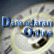##Debt Ratio Regression: January 2015

Variables used in the regression

1. Debt Ratio = Debt/ (Market Value of Equity + Debt): If you can get market value of debt, use it. Else, use book value of debt.
2. Pre-tax ROC = EBIT/ (Book Debt + Book Equity - Cash)
3. Expected growth rate in revenues - next 2 years= You can use expected or even historical earnings growth, if you don't have a revenue growth forecast
4. EBITDA/ Enterprise Value = EBITDA/ (Market Value of Equity + Book value of Debt - Cash)

US Regression

 Model Summarya Model R R Square Adjusted R Square Std. Error of the Estimate 1 .429b .184 .183 12.67747 a. Broad Group = United States b. Predictors: (Constant), Return on Capital (ROC or ROIC), EBITDA‎/EV, Expected growth in revenues - Next 2 years

 Coefficientsa,b,c Model Unstandardized Coefficients Standardized Coefficients t Sig. B Std. Error Beta 1 (Constant) .236 .008 30.324 .000 Expected growth in revenues - Next 2 years -.023 .014 -.032 -1.613 .107 EBITDA‎/EV .548 .071 .152 7.672 .000 Return on Capital (ROC or ROIC) -.367 .017 -.398 -21.034 .000 a. Broad Group = United States b. Dependent Variable: Market Debt to Capital Ratio c. Weighted Least Squares Regression - Weighted by Market Cap (in US \$)

Global Regression

 Model Summary Model R R Square Adjusted R Square Std. Error of the Estimate 1 .472a .222 .222 10.43826 a. Predictors: (Constant), Return on Capital (ROC or ROIC), Expected growth in revenues - Next 2 years, EBITDA‎/EV

 Coefficientsa,b Model Unstandardized Coefficients Standardized Coefficients t Sig. B Std. Error Beta 1 (Constant) .278 .003 81.203 .000 Expected growth in revenues - Next 2 years -.008 .005 -.014 -1.604 .109 EBITDA‎/EV .396 .023 .147 16.873 .000 Return on Capital (ROC or ROIC) -.475 .009 -.433 -50.654 .000 a. Dependent Variable: Market Debt to Capital Ratio b. Weighted Least Squares Regression - Weighted by Market Cap (in US \$)

• How do I use this regression?

Assume that you want to estimate the market debt ratio for a firm with the following characteristics, using the US regression

EBITDA/ Enterprise Value = 8%

Pre-tax return on capital = 20%

Expected growth rate in revenues = 15%

• Predicted Value

Expected Debt Ratio= .236 -.023 (.15) + .548 (.08) -0.367 (.20) = .203 or 20.30%

If your predicted value is less than zero, your predicted debt ratio is zero.